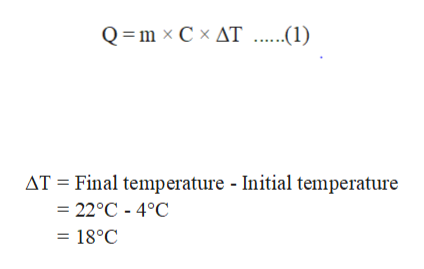# A large bottle containing 883 g of water at 4 °C is removed from the refrigerator. How many kilojoules are absorbed to warm the water to room temperature of 22 °C?

Question
34 views

A large bottle containing 883 g of water at 4 °C is removed from the refrigerator. How many kilojoules are absorbed to warm the water to room temperature of 22 °C?

check_circle

Step 1

Given that,
Mass of water is 883 g.

The initial temperature is 4˚C.

The final temperature is 22˚C.

It is well known that the specific heat of water is 4.184 J/g˚C.

The amount of heat absorbed can be calculated by the use of heat equation given below:

Here,

Q is the amount of heat absorbed.

m is the mass of the substance.

ΔT is the change in temperature.

Change in temperature can be calculated as follows:help_outlineImage TranscriptioncloseQ =m x C x AT...1 AT Final temperature - Initial temperature =22°C - 4°C = 18°C fullscreen
Step 2

Substitute the given values in the equation (1) to calculate the amount of heat absorbed ...

### Want to see the full answer?

See Solution

#### Want to see this answer and more?

Solutions are written by subject experts who are available 24/7. Questions are typically answered within 1 hour.*

See Solution
*Response times may vary by subject and question.
Tagged in

### Chemistry